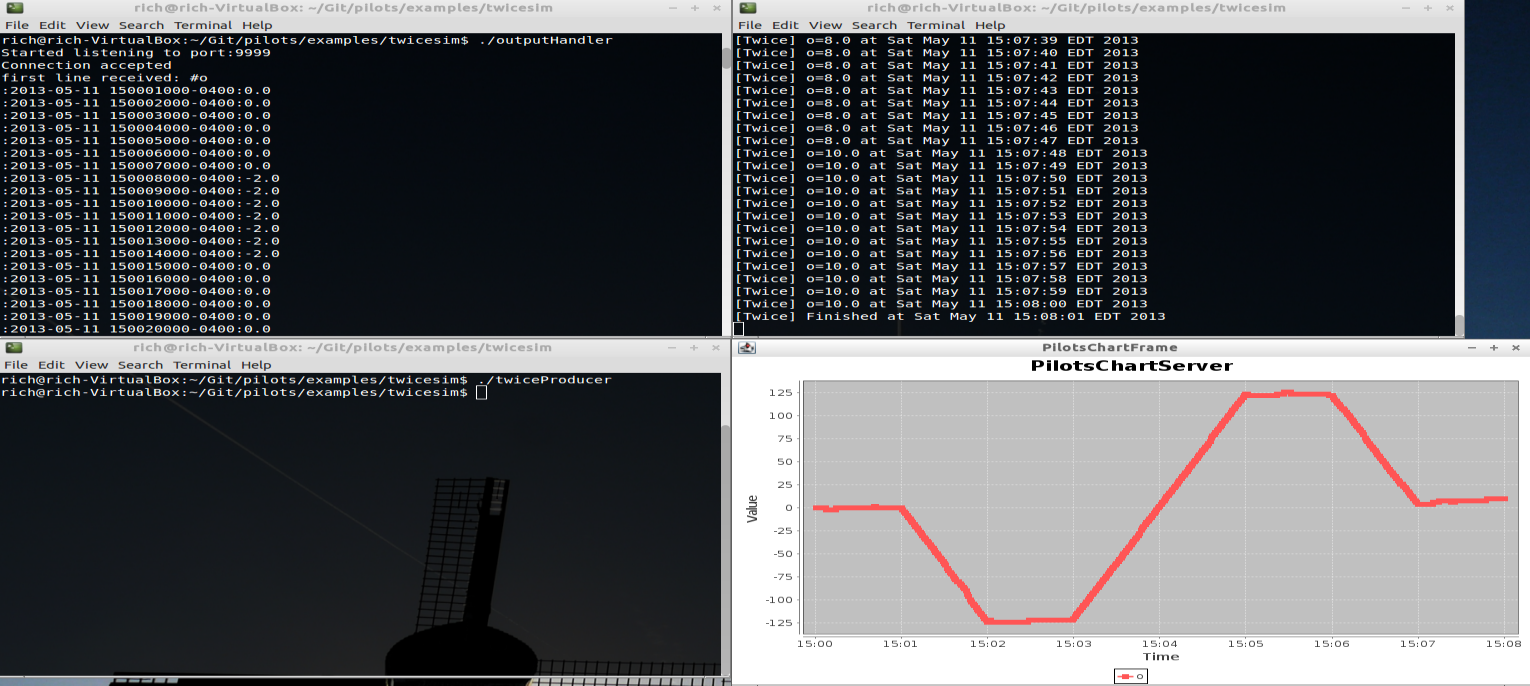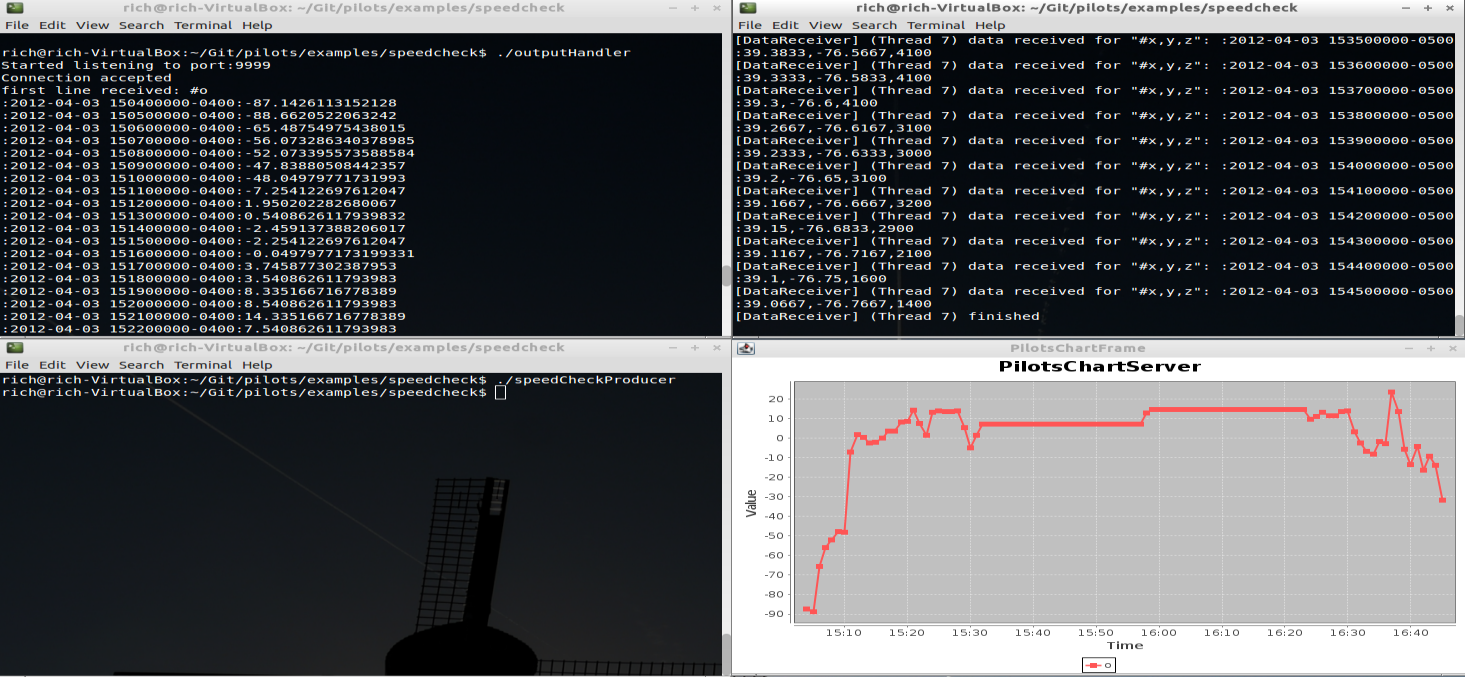# PILOTS: Examples Tutorial

PILOTS home     WCL home
Tutorial index     (Basic ExamplesProgrammingRunningLearning Model OverviewLinear Regression Example )

# Introduction

This section of the tutorial deals with running the examples from the associated publications. The source code for these examples can be found in \$PILOTS_HOME/examples; each example comes precompiled in the PILOTS distribution. Please note that each example comes in two flavors: corrected and uncorrected. The instructions below are for running uncorrected examples. In order to run the corrected version of the examples simply run the script that ends with "Correct."
The examples include:

1. Twice (real-time mode)
• Two input streams (a,b) where, at any given moment, the value of the data on input stream b is twice the value of the data on input stream a. The output stream o, computed as b-2*a, should continuously produce zero under normal circumstances.

2. Twice (simulated mode)
• Similar to the real-time mode, but the input data is generated via a file (in \$PILOTS_HOME/11-May-2013_Twice) using a simulated time counter.

3. SpeedCheck
• A simulated flight-monitoring application that uses real data recorded on April 3rd, 2012 during a flight from Albany, NY to Tipton, MD. The application monitors the air speed, wind speed, and ground speed of the moving object to detect when there is a failure in any one (or a combination) of the sensors.

Each of these examples make use of external software components provided in the PILOTS distribution. These will be described in more detail in the running tutorial.

# Twice (real-time mode)

Twice is a very basic streaming application with two input streams a and b. At every given point in time, the value on stream b should be twice the value on stream a. We use PILOTS to easily produce this application. Furthermore, we were able to observe the PILOTS application to produce error correcting code. This is the first example that is used to exemplify error signatures.

Before running the executable scripts, complete the following steps for compiling the plt file:

1. Run the following command to compile the PILOTS program to Java source code:
``\$ plc Twice.plt ``

2. Run the following command to compile the generated Java source code:
``\$ javac Twice.java ``

Finally, in order to run this example, open four identical terminals looking at the \$PILOTS_HOME/examples/twice/ directory. Be sure that your classpath includes \$PILOTS_HOME/lib/* in each of these terminals. Running the PILOTS application is a three step process. First the data sink (in this case, an external software component to visualize output) must be initialized. Next the application and finally all data sources. In this example the data source is an external software component. We have provided scripts that make executing these examples more convenient. The three steps described above are summarized as follows:
1. On one terminal, run the outputHandler script. This will initialize the data sink and wait for the PILOTS application to produce an output stream.

2. On the next terminal, run the twice script. This will initialize the PILOTS application, connect with the output handler's socket, and start listening for input data.

3. On the last two terminals, run the twiceProducerA and twiceProducerB scripts (respectively). Try to make sure that these two processes are initialized as close as possible. Starting one a long while after the other will cause an out of sync error in the PILOTS application.

Here is a screenshot of the Twice application in action:In order to simulate a failure in one of the streams, kill the data producing process. For example to simulate a failure in stream b you can activate the terminal that is running twiceProducerB and kill the proces (using CTRL+C). These errors produce characteristic patterns which we describe using error signatures. Error signatures will be discussed more in the programming tutorial

# Twice (simulated mode)

This example is functionally equivalent to the real-time version of Twice. However in the simulated mode, all data is generated from a file. Thus all data is known ahead of time, and there is no waiting for streams to produce new data.

Before running the executable scripts, complete the following steps for compiling the plt file:

1. Run the following command to compile the PILOTS program to Java source code:
``\$ plcsim Twice.plt ``

2. Run the following command to compile the generated Java source code:
``\$ javac Twice.java ``

Finally, running the simulated version of Twice is slightly different than running the real-time version. Only three terminals need to be opened, all directed to \$PILOTS_HOME/examples/twicesim.
1. On the first terminal, run the outputHandler script.

2. On the second terminal, run the twicesim script.

3. On the third terminal, run the twiceProducer script. This will send all of the data from the files in \$PILOTS_HOME/data/11-May_2013 to the PILOTS application.

4. Finally, to initiate the simulation activate the window that is running the twicesim script and press ENTER.

Here is a screenshot of the simulated Twice application after execution:Note that failures are simulated by the input data themselves. The files in \$PILOTS_HOME/data/11-May-2013_Twice were generated programmaticaly to exhibit certain errors at certain times (see the publication regarding error detection and correction for details).

# SpeedCheck

This example is a more practical motivating example. Real data was recorded during an actual flight (see Quest paper for details). This data includes air speed (measured from plane's sensor), ground speed (measured from GPS), and wind speed (measured from weather reports). Using the principles of relative forces we are able to derive equations for each of these speeds in terms of the other two. The point of this example is to show that under certain erroneous conditions, such as a pitot tube (airspeed sensor) freezing, it is possible to recompute erroneous data from redundant counterparts. This application also uses simulated mode, the data can be found in \$PILOTS_HOME/data/03-Apr-2012-KALB-KFME. Three terminals need to be opened all pointing to \$PILOTS_HOME/examples/speedCheck.

Before running the executable scripts, complete the following steps for compiling the plt file:

1. Run the following command to compile the PILOTS program to Java source code:
``\$ plcsim SpeedCheck.plt ``

2. Run the following command to compile the generated Java source code:
``\$ javac SpeedCheck.java ``

Finally, use the following steps to execute the example:
1. On the first terminal, run the outputHandler script.

2. On the second terminal, run the speedCheck script

3. On the third terminal, run the speedCheckProducer script. Then, in the terminal window that is running the speedCheck script, press ENTER.

Here is an screenshot of the SpeedCheck application after execution:In order to simulate different errors (such as airspeed sensor failure), replace the speedCheckProducer script with one of the other speedCheckProducer_* scripts.

Tutorial index     (Basic ExamplesProgrammingRunningLearning Model OverviewLinear Regression ExampleBayes Classifier Example)
PILOTS home     WCL home